# How to calculate the absolute difference between two values/times in Excel?

The usage of absolute numbers to distinguish between occurrences like "Decline," "Boost," and "Payment," among other things, is rather prevalent among users. When just the difference between the two numbers is required or requested in a spreadsheet, the Excel absolute difference between the two values is provided. The ABS function in Excel will return the absolute value of any difference or number that is provided. To calculate the absolute difference between two values, we may make use of a variety of different formulae, including IF, MAX, and MIN, as well as a VBA Custom Function.

In layman's terms, the absolute value of a number is the distance that number is from zero on a number line, independent of the direction in which the number is placed on the number line.

## Step 1

Determine the absolute difference between the two students, A and B, using this example.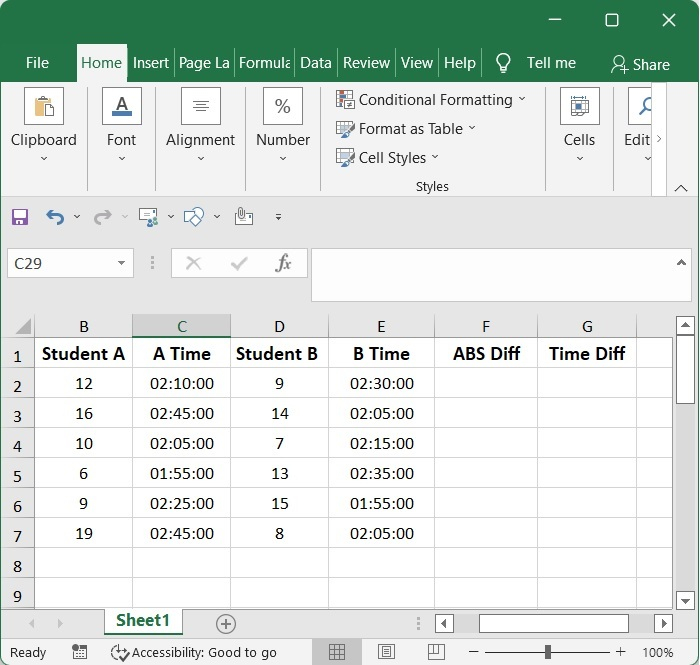## Step 2

To compute the absolute differences, all you need is one of the following formulae, which you should copy and paste into the blank cell in which you want the result to be placed.

=ABS(A2-B2)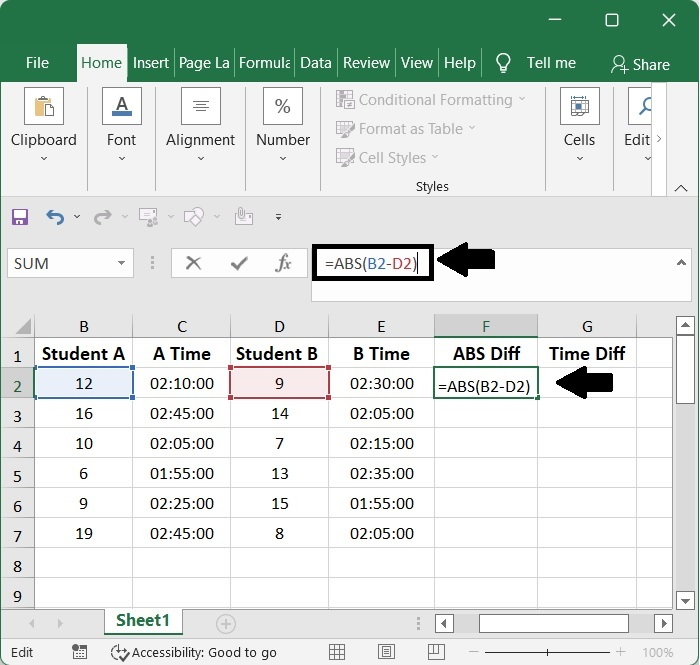## Step 3

Numbers are acceptable for use as the parameter in the ABS function. We can easily determine the absolute difference between two numbers by first calculating the difference between the numbers using the Arithmetic Operator and subsequently employing the ABS function.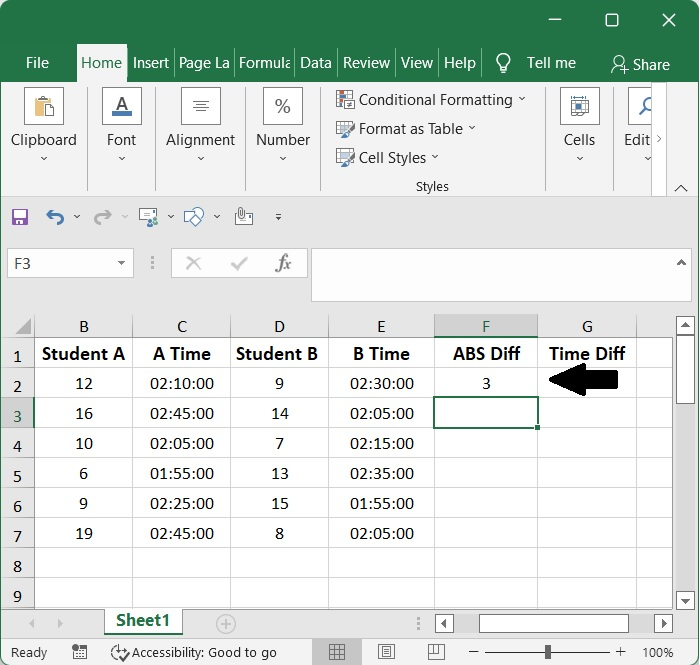## Step 4

Afterwards, you should move the fill handle over the cells that are required by this formula after pressing the Enter key.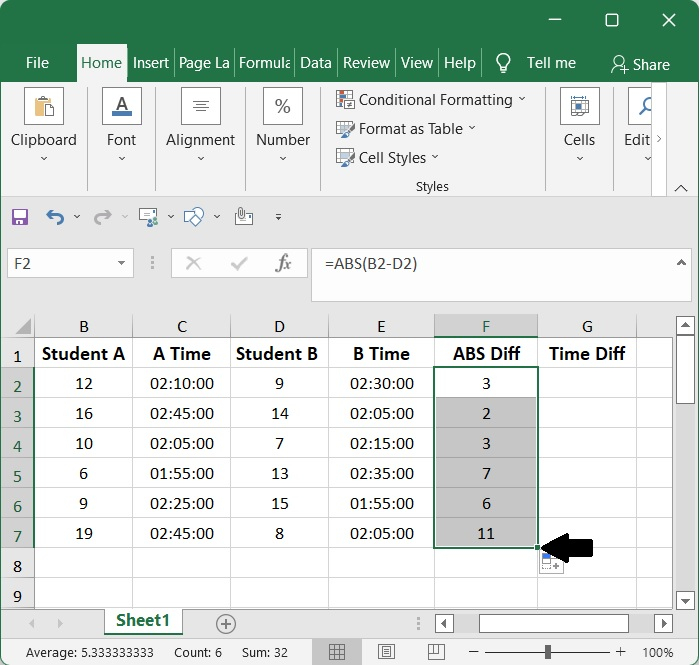## Step 5

The only thing you need to calculate the time differences absolute is one of the following equations, which you should copy and paste into the empty cell in which you desire to keep the result of the calculation. This is the only thing you need to do in order to compute the time differences absolute.

=ABS(C2-E2)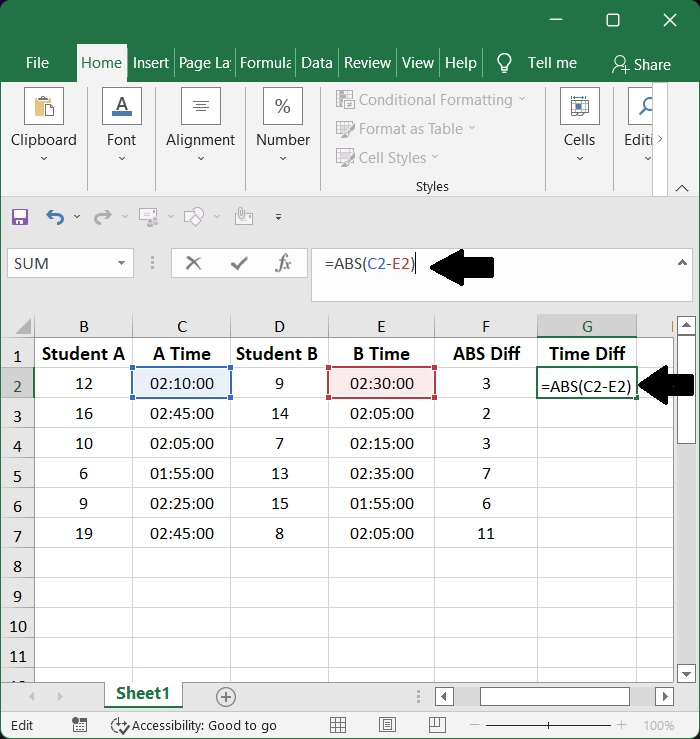## Step 6

You have just one option, which is to apply a formula, and then format the numbers as time, if you wish to determine the absolute difference between two times.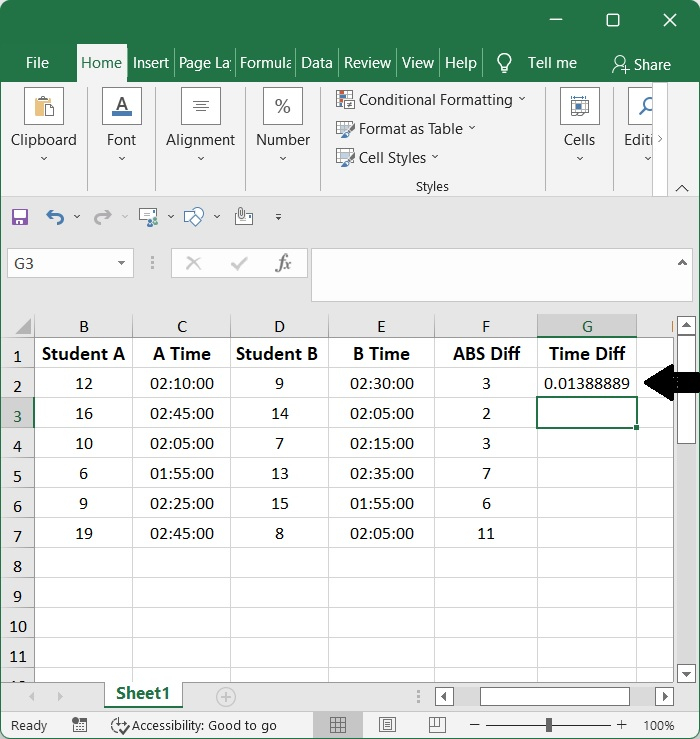## Step 7

After clicking the Enter key, you should then slide the fill handle over the cells that are needed by this formula. This should be done after the formula has been applied.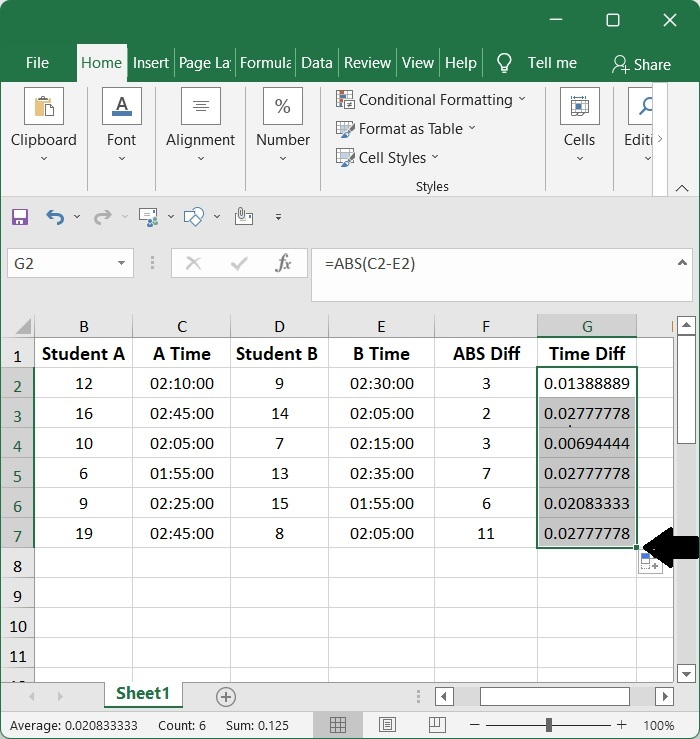## Step 8

In order to translate this value into a certain amount of time, go to the Home tab, look for the Cell, and then click Format Cells in the Format drop-down menu.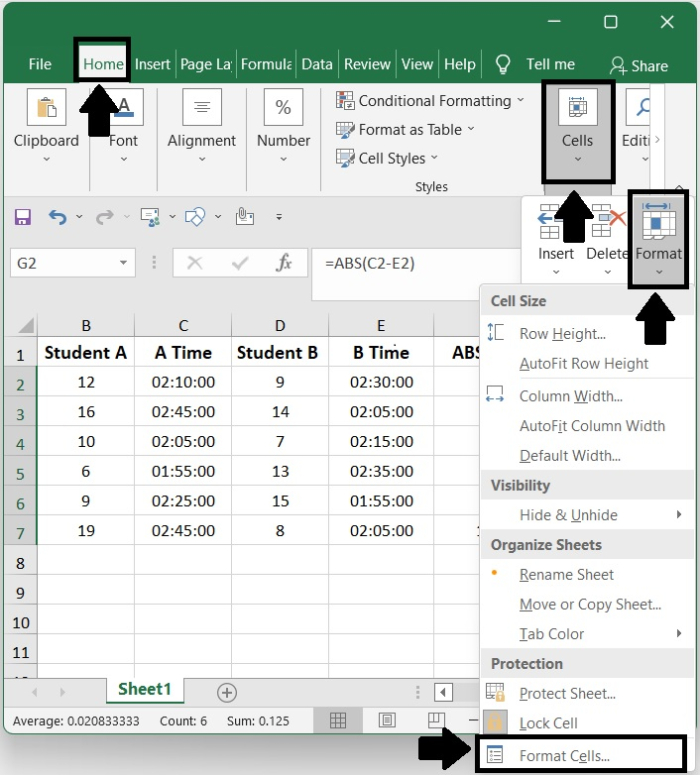## Step 9

The Format Cells pop-up window will then appear. Click ok after opening the window, selecting 13:30:55 in the Type area of the window, and opening the Number window.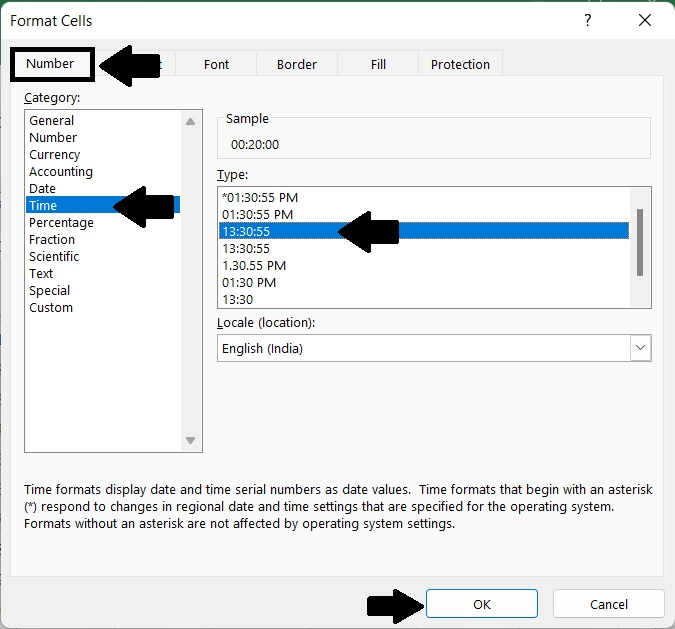## Step 10

At long last, I've calculated the absolute time difference between two values/times.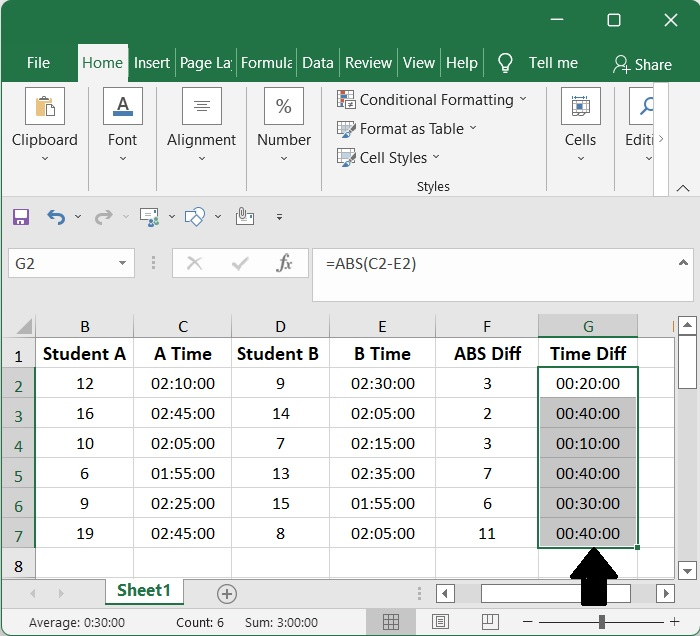## Conclusion

In this tutorial, we used a simple example to demonstrate how you can calculate the absolute difference between two values/times in Excel.

Updated on: 06-Feb-2023

649 Views